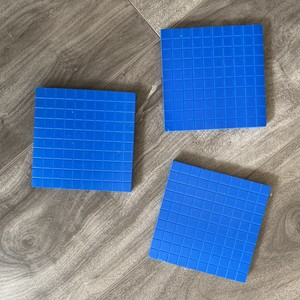Subtraction
Problem Solving
Place Value
Mystery
100

12 + 3 =

15

100

12 - 6 =

6

100

How many tens and hundreds are in

300 ones.

30 tens

3 hundreds

100What number does the model above represent?

256

100

Write the number 175 in expanded form.

100+70+5

200

13 + 7 =

20

200

18 - 7 =

11

200

Mrs. Densford has a bag of hershey kisses.    If there is a 7 in the tens place, a 2 in the ones place, and a 4 in the hundreds place, how many hershey kisses does Mrs. Densford have?

427

200

Write down which digit is in the tens place.

548

4

200

Skip count by 5s: 15,20,_____,30,_____,40

15,20,25,30,35,40

300

13 + 17 =

30

300

23 - 9 =

14

300

There were 49 butterflies in a greenhouse.    Some flew away.   There are still 33 in the greenhouse.  How many flew away?

16

300

What is the value of the 8 in 857?

800

300

Use base ten drawings to represent the following number:308

Students should have 3 flats, 0 rods, and 8 units drawn on their white boards.

400

26 + 38 =

64

400

36 - 29 =

7

400
There were 45 snow cones sold at the festival.  They also sold some cotton candy.   If 71 food items were sold in all how many were cotton candy.

26

400

The number of people at the zoo has a 3 in the ones place, a 6 in the hundreds place, and a 2 in the tens place. Write the number that represents the people at the zoo and draw a base ten representation for it.

623

6 flats,2 rods, and 3 units

400

What word means the answer to a subtraction problem?

Difference

500

72 + 28 =

100

500

51 - 33 =

18

500

Jace has 24 pokemon cards and the rest of his cards are baseball cards.   If he has 33 cards total, how many are baseball cards.

9

500

Write how many hundreds,tens,and ones for the model below.______hundreds=________tens=________ones

3 hundreds=30 tens= 300 ones

500

Do you have to regroup ones as tens to do the following problem? Write yes or no 40+29

No

Click to zoom